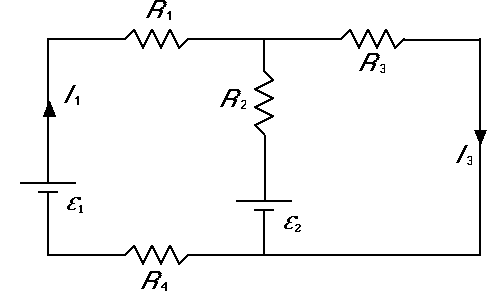# Kirchoff's Equation w/ 2 Batteries and 4 resistors

wizzleman

## Homework Statement

The circuit in the figure is composed of two batteries (ε1 = 9.0 V and ε2 = 5.0 V) and four resistors (R1 = 110.0 Ω, R2 = 40.0 Ω, R3 = 30.0 Ω, and R4 = 50.0 Ω) as shown.

1). What is the current I1 which flows through R1?

2). What is the current I3 which flows through R3?## Homework Equations

I used

ε1-R1I1+I2R2-R4I1-ε2=0

and

ε1-R1I1-R3I3-R4I1=0

## The Attempt at a Solution

I've tried plugging in variables and attempting to solve for I3 but it's not the correct answer.

Staff Emeritus
Homework Helper
Gold Member

## Homework Statement

The circuit in the figure is composed of two batteries (ε1 = 9.0 V and ε2 = 5.0 V) and four resistors (R1 = 110.0 Ω, R2 = 40.0 Ω, R3 = 30.0 Ω, and R4 = 50.0 Ω) as shown.

1). What is the current I1 which flows through R1?

2). What is the current I3 which flows through R3?## Homework Equations

I used

ε1-R1I1+I2R2-R4I1-ε2=0

and

ε1-R1I1-R3I3-R4I1=0

## The Attempt at a Solution

I've tried plugging in variables and attempting to solve for I3 but it's not the correct answer.
Hello wizzleman. Welcome to PF !

What you have looks correct.

You need another equation --- or a method to avoid using I2.

How is I2 related to I1 and I3 ?

wizzleman
Hello wizzleman. Welcome to PF !

What you have looks correct.

You need another equation --- or a method to avoid using I2.

How is I2 related to I1 and I3 ?

For the junction equation is I1= I2+I3 ?

Staff Emeritus
Homework Helper
Gold Member
For the junction equation is I1= I2+I3 ?
To make your equation, ε1-R1I1+I2R2-R4I1-ε2=0, correct, you need I1 + I2 = I3 .

wizzleman
To make your equation, ε1-R1I1+I2R2-R4I1-ε2=0, correct, you need I1 + I2 = I3 .

Thanks for all the help so far, but I still can't get the answer.

I decided to make three loops

Left loop was ε1-R1I1+R2I22-R4I1=0

Right loop was ε2-R2I2-R3I3=0

And the whole loop was ε1-R1I1-R3I3-R4I1=0

Junction was I1=I2+I3

Solving through and using elimination I got up to 8V-320I1+80I2=0

How am I supposed to use the junction equations in here?

Staff Emeritus
Homework Helper
Gold Member
Thanks for all the help so far, but I still can't get the answer.

I decided to make three loops

Left loop was ε1-R1I1+R2I22-R4I1=0

Right loop was ε2-R2I2-R3I3=0

And the whole loop was ε1-R1I1-R3I3-R4I1=0

Junction was I1=I2+I3

Solving through and using elimination I got up to 8V-320I1+80I2=0

How am I supposed to use the junction equations in here?
Two loop equations plus the junction equation give three equations in three unknowns. That is enough to solve for the three unknowns. The addition loop equation is not independent of the other two.

Your junction equation is inconsistent with your Left Loop & Right Loop equations. Both loop equations are consistent with I2 flowing in the upward direction. That implies that I[1/SUB] + I2 = I3, as I said in post #4.

Solve that for I2: I2 = I3 - I1 .

Plug that into the equation for the right loop.

ε2 - R2(I3 - I1) - R3I3=0

This can be written:
ε2 + R2I1 - (R2+R3)I3 = 0

Use that with the "whole loop" equation:
ε1 - (R1 + R4)I1 - R3I3 = 0

Last edited:
Homework Helper
Gold Member
The circuit is equivalent to this one. You can apply KCL to the bottom node to get an equation for the voltage across R3. Call that VR3

I3 + I2 + I1 = 0

VR3/R3 + (VR32)/R2 + (VR31)/R1+4 = 0

Solve for VR3

It's then easy to calculate all three currents.

Edite to make it clearer.

#### Attachments

wizzleman
Thanks for all the help guys, I figured out the answers for I1 and I3.